# Study Notes For Filter Circuit & Coupled Circuit Notes | Study Network Theory (Electric Circuits) - Electrical Engineering (EE)

## Electrical Engineering (EE): Study Notes For Filter Circuit & Coupled Circuit Notes | Study Network Theory (Electric Circuits) - Electrical Engineering (EE)

The document Study Notes For Filter Circuit & Coupled Circuit Notes | Study Network Theory (Electric Circuits) - Electrical Engineering (EE) is a part of the Electrical Engineering (EE) Course Network Theory (Electric Circuits).
All you need of Electrical Engineering (EE) at this link: Electrical Engineering (EE)

FILTER CIRCUITS
Circuits with a response that depends upon the frequency of the input voltage are known as filters. Filter circuits can be used to perform a number of important functions in a system. Although filters can be made from inductors, resistors, and capacitors most filter circuits are based upon Op-Amps, Resistors, and Capacitors.

Filter types and characteristics

• Low-pass filters: allow any input at a frequency below a characteristic frequency to pass to its output unattenuated or even amplified.
• High-pass filters: allow signals above a characteristic frequency to pass unattenuated or even amplified.
• Band-pass filters: allow frequencies in a particular range to pass unattenuated or even amplified.

The transfer function of a filter is characterized by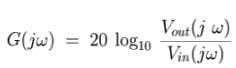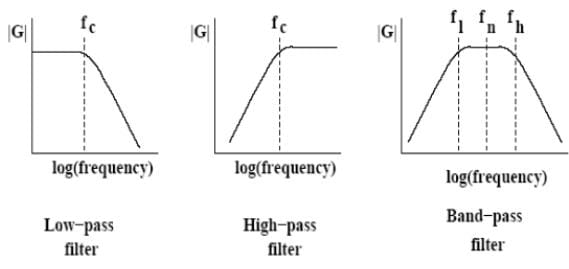THE BUTTERWORTH FILTER
The figure below shows the magnitude response of a Butterworth filter.This filter exhibits a monotonically decreasing transmission with all transmission zeroes at ω=∞, making it an all pole The magnitude function for a Nth-order Butterworth filter with a passband edge ω is given by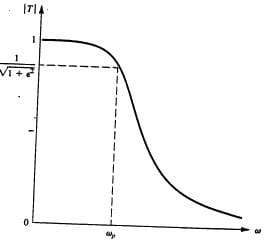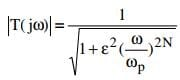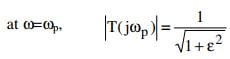The parameter ε determines the maximum variation in passband transmission, AMAX (i.e, passband ripple).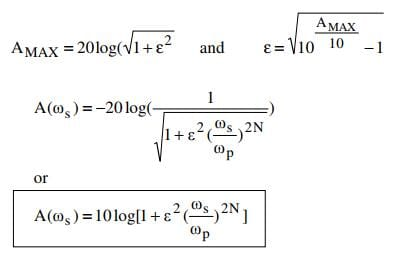The last equation is used to determine the filter order required, which is the lowest value of N that yields A(ωs) ≥ AMIN.

Applications of Filters:
(a) Anti-Alias Filtering-Aliasing is a phenomenon that arises because all digital systems can only represent a signal at particular instances in time. A schematic diagram showing signals of two different frequencies which are indistinguishable when they are sampled at a finite number of points.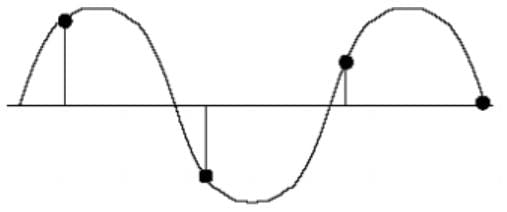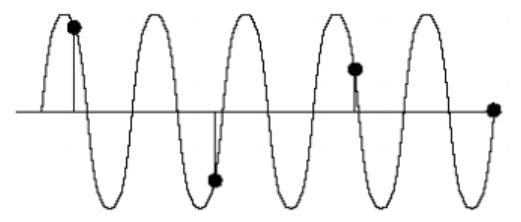If the continuous input signal contains no frequency components higher than fc, then it can be completely recovered without distortion if it is sampled at the rate of at least 2fc samples per second.Thus before a signal is sampled into a digital system the designer must determine the maximum frequency, fmax, in the input signal that will contain useful information. A filter must then be designed which attenuates all frequencies above fmax, prior to sampling by the ADC at a frequency of at least 2*fmax,. This type of anti-aliasing filter must be present in any well designed system that includes an analogue to digital converter.
(b) Noise Rejection The quality of a signal in the presence of noise is most often specified by the signal-to-noise ratio (SNR) which is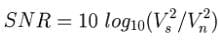where  Vs is the rms value of the signal and Vn is the rms value of the noise.
(c) Interference Rejection: Although interference can be minimised by careful design it is sometimes necessary to also employ filters. Often the dominant source of interference is the mains power supply and so it can be necessary to use a filter to attenuate the frequency of the local mains power supply, 50 Hz in the UK, and/or some of its harmonics.
Note. Using a band-pass filter with a characteristic frequency of 500Hz it is possible to reduce the amount of interference by a factor of 400 without attenuating the signal at 500Hz.

The document Study Notes For Filter Circuit & Coupled Circuit Notes | Study Network Theory (Electric Circuits) - Electrical Engineering (EE) is a part of the Electrical Engineering (EE) Course Network Theory (Electric Circuits).
All you need of Electrical Engineering (EE) at this link: Electrical Engineering (EE)Use Code STAYHOME200 and get INR 200 additional OFF

## Network Theory (Electric Circuits)

23 videos|21 docs|27 tests

Track your progress, build streaks, highlight & save important lessons and more!

,

,

,

,

,

,

,

,

,

,

,

,

,

,

,

,

,

,

,

,

,

;